# KSEEB Solutions for Class 7 Maths Chapter 9 Rational Numbers Ex 9.1

Students can Download Chapter 9 Rational Numbers Ex 9.1, Question and Answers, Notes Pdf, KSEEB Solutions for Class 7 Maths, Karnataka State Board Solutions help you to revise complete Syllabus and score more marks in your examinations.

## Karnataka State Syllabus Class 7 Maths Chapter 9 Rational Numbers Ex 9.1

Question 1.
List five rational numbers between:
a) -1 and 0
Solution:we see that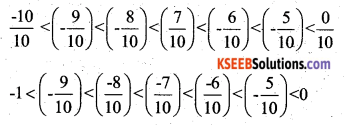∴ Five rational numbers between -1 and 0 are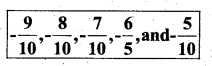ii) -2 and -1
Solution:we see that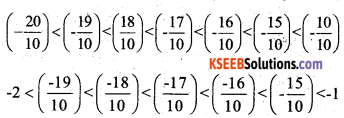∴ Five Rational Numbers between -2 and -1 are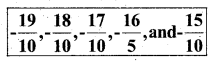iii)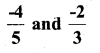Solution:we see that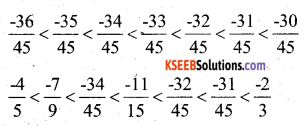Thus five rational numbers between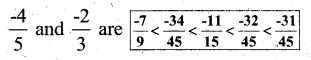iv)Solution:we see thatThus 5 Rational Numbers in between $$\frac{1}{2}$$ and $$\frac{2}{3}$$ are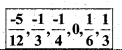Question 2.
Write four more rational numbers in each of the following patterns :
i)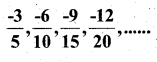Solution:Thus we came to know the pattern in the above numbers ie., both numerator and denominator should be multiplied by same whole number.
∴ The four more rational numbers would be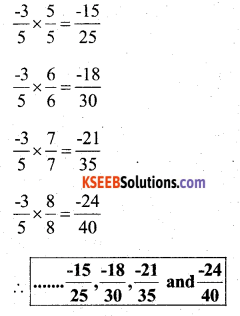ii)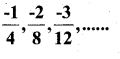Solution:Thus we observe the pattern in these numbers.
The other numbers would be,iii)Solution: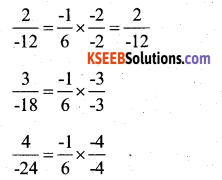Thus we observe the pattern in these numbers. The other numbers would be,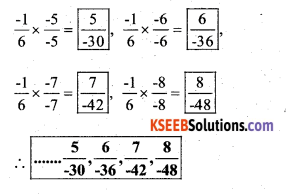iv)Solution:Thus we observe the pattern in these numbers
The other numbers would be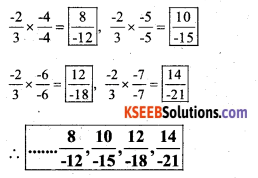Question 3.
Give four rational numbers equivalent to :
i) $$\frac{-2}{7}$$
Four rational numbers equivalent to $$\frac{-2}{7}$$ are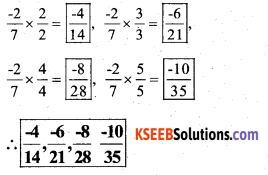ii) $$\frac{5}{-3}$$
Four rational numbers equivalent to $$\frac{5}{-3}$$ are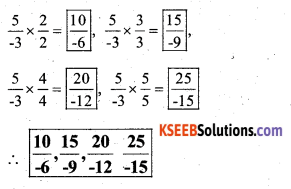iii) $$\frac{4}{9}$$
Solution:
Four rational numbers equivalent to $$\frac{4}{9}$$ areQuestion 4.
Draw the number line and represent the following rational numbers on it :
i) $$\frac{3}{4}$$
Solution: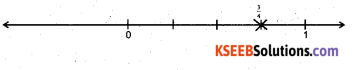ii) $$\frac{-5}{8}$$
Solution: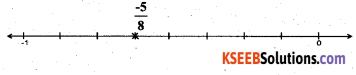iii) $$\frac{-7}{4}$$
Solution:iv) $$\frac{7}{8}$$
Solution: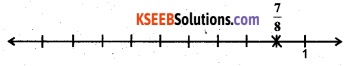Question 5.
The points P, Q, R, S, T, U, A and B on the number line are such that, TR = RS = SU and AP = PQ = QB. Name the rational numbers represented by P, Q, R and S.
Solution:The rational number represented byThe rational number represented byThe rational number represented byThe rational number represented byThe rational number represented by P Q R S is equal torespectively.Question 6.
Which of the following pairs represent the same rational number ?
i)Solution:
$$\frac{-7}{21}$$ is negative rational number and $$\frac{3}{9}$$ is a positive Rational Number. Therefore this pair does not represent the same rational number.

ii)Solution: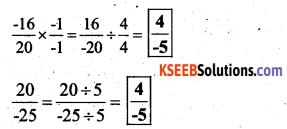∴ The above pair of numbers represent the same rational number.

iii)Solution:∴ The above pair of numbers represent the same rational number.

iv)Solution:∴ The above pair of numbers represent the same rational number.

v)Solution: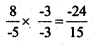∴ The above pair of numbers represent the same rational number.vi)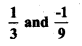Solution:
$$\frac{1}{3}$$ is a positive rational number and $$\frac{-1}{9}$$ is a negative Rational Number.
Therefore this pair does not represent the same rational number.

vii)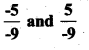Solution: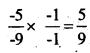is a positive rational number and $$\frac{5}{-9}$$ is a negative rational number.

Question 7.
Rewrite the following rational numbers in the simplest form :

i) $$\frac{-8}{6}$$
The H. C. F of 6 and 8 is 2.
Then its simplest form would be,ii) $$\frac{25}{45}$$
The H. C. F of 25 and 45 is 5.
Then its simplest form would be,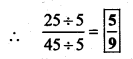iii) $$\frac{-44}{72}$$
The H. C. F of 44 and 72 is 4.
Then its simplest form would beiv)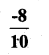The H. C. F of 8 and 10 is 2.
Then Its simplest form would be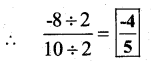Question 8.
Fill in the boxes with the correct symbol out of >, <, and =.

i)Solution:
$$\frac{-5}{7}$$ is a negative rational number
$$\frac{2}{3}$$ is a positive rational number.ii)Solution:
To make the equal denominatoriii)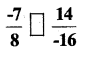Solution: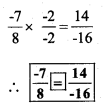iv)Solution:v)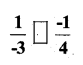Solution:vi)Solution: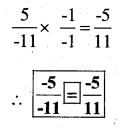vii)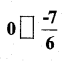Solution:
0 is greater than the negative numbers,Question 9.
Which is greater in each of the following

i)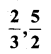Solution:
L.C.M of 3 & 2 is 6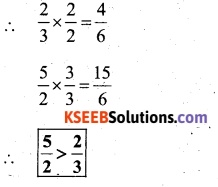ii)L. C. M of 6 and 3 is 6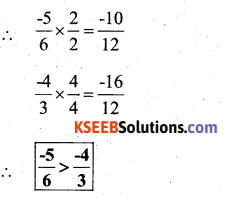iii)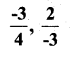Solution:
L. C M of 4 and 3 is 12iv)Solution: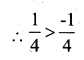(∵ All positive numbers are greater than all negative numbers.)

v)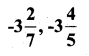Solution: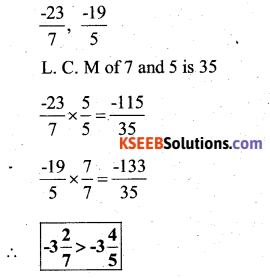Question 10.
Write the following rational numbers in ascending order :
i)-3 < -2 < -1
∴ Ascending Order is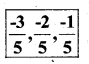ii)Solution:
L. C. M of 3 & 9 is 9iii)Solution: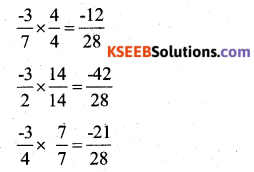Denominator same, So the numerators are
-42 < -21 < -12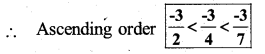error: Content is protected !!